Well, creating formulas are not everyone's cup of tea, but creating them on Stackby is easy. Having said, there are a few pre-requisites and norms that you need to be aware of before you start creating those simple or complex formulas on Stackby. In this article, we will take a look at these norms and pre-requisites. But first, let us discuss the different formats when it comes to formulas:

Integer

String (Text)

Decimal

Date

Currency

Percentage

## Integer

Integers are nothing but a set of numbers without fractions. These could be negative or positive.

You need to select this format when applying any formula with numbers. Usually, these are used in basic mathematical formulas of addition, subtraction, division or multiplication. The only norms that you need to remember with these are:

• Adding two positive integers will always result in a positive integer.
• Adding two negative integers will always result in a negative integer.
• Adding one positive and one negative integer will result in a positive number if a positive integer is a greater and negative number if a negative integer is greater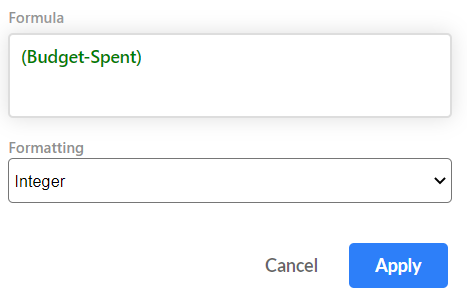## String (Text)

Strings refer to sequences of text and characters. You can use a CONCAT or Concatenate formula to bring together text and characters in different fields.

• A CONCAT formula needs “String (text)” for its proper formatting
• You also need to use String (text) in the formatting field if your formula uses emoji or IF in them.
• A date formula, you need to select “Date” from the formatting drop-down menu
• If you are looking at getting the calculation result as a percentage, then make sure you select “percentage” from the format menu.

There are other conveniences too:

You can use the currency formatting if the formula fields are currencies and you need to see the result in currency.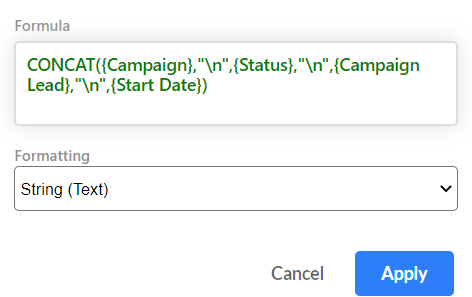## Decimals

If you love your decimals, select it in the formatting menu so that figures are not rounded off (you can also select this as an option in currency and percentage if you want the actual calculation and not the rounded-off value).

This could be anything from your percentages to currency or simple Math formulas.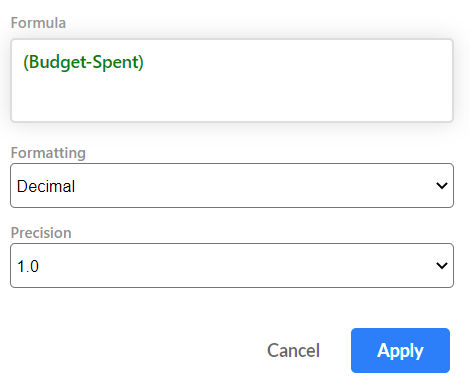## Date

If you are using a date formula -- a formula that needs to have a column with Date and time property in them - you select Date from the formatting menu.

Here are a few examples:

TONOW (), FROMNOW(), CREATED_TIME, DATE_ADD(), DATE_SUB(), MONTH(), YEAR(), DAY()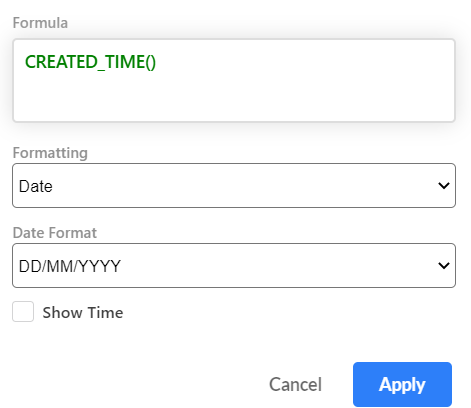## Currency

You must select Currency as a format if you need monetary values in the formula results (say, profit, Sales Price, Cost Price, etc.).

Note:  We have Dollars (\$) as the default currency. However, if you need to see the values in a different currency, please add in the relevant currency symbol in the "currency symbol" field and it will automatically show the values in that currency.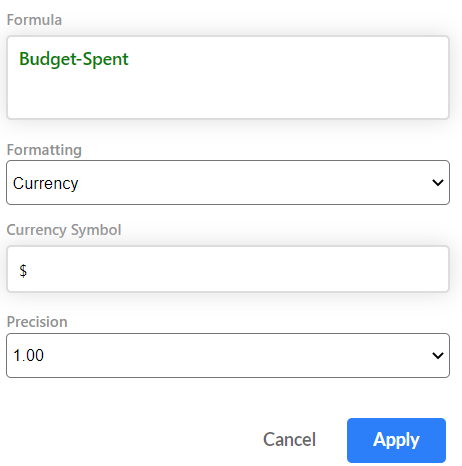## Percentage

If you require your formula results in percentages, please make sure to select "percentage" in the format field. This will automatically add a % symbol to formula results. You also have the option of choosing decimals for percentages.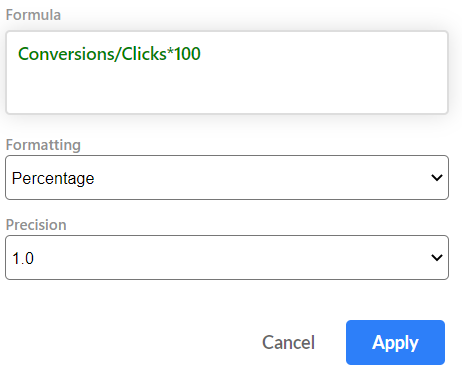Oh! One more thing! A gentle reminder that all formulas work at the Column level and not at the cell level. For those of you who are Excel or spreadsheet fans, this could be a different path for you, but we promise it is an easy one. Also, if you are typing out the name of your fields, then make sure you put in the correct name to the last dot and space. If the field names are made up of two words and have space in between, put them in curly brackets so that Stackby knows that is the exact field it needs to consider to calculate.